# Basic Fraction Worksheets

Print and use any of the fraction strips, fraction circles, fraction games, and fraction worksheets on this page with your students.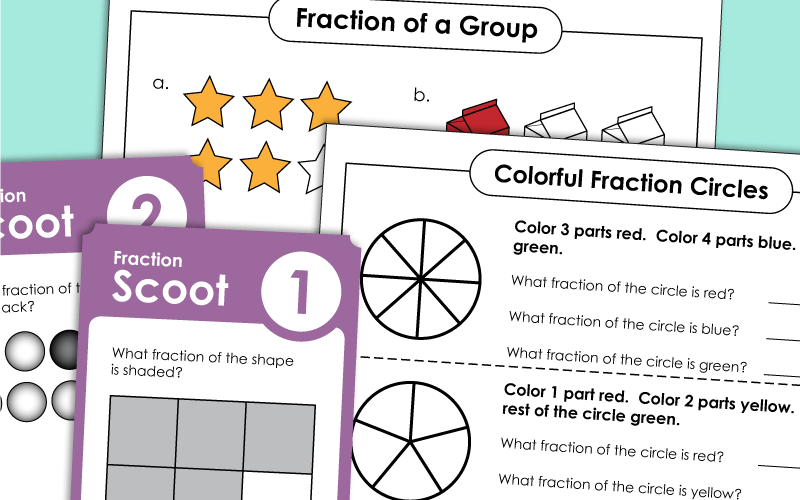## Basic Fraction Worksheets

Color the circle according to the directions, then write the fractions of the shaded parts.
Tell whether each shape is divided into halves, thirds, fourths, sixths or eighths.
Students will divide rectangles into equal parts and color according to the instructions.
Students divide circles into equal parts and then color according to the instructions.
Answer these fraction thinking problems. Students must find different ways to divide shapes, determine which shapes are divided into fractions, and complete the fractions of a set drawings.
This file has 30 task cards that can be used for learning centers, on your whiteboard's document camera, or for small group instruction.

## Fraction Cut & Glue

Match each fraction with the correct shape in this cut-and-paste activity.
Students color the shape on their fraction flag according to the key. Then cut out the flags. Teachers can hang the colorful pennants on a string across the classroom.
Cut, color, and fold the origami cootie catcher (a.k.a. fortune teller). Then play the game to review basic fractions.

## Fractions of ShapesWorksheets

Fill in the missing numerator or denominator for each fraction.
Part 1: Shade each shape to show the fraction given. Part 2: Tell what fraction is shown. Part 3: Divide the square into quarters and shade 3/4.
Tell what fraction of each shape is shaded.

## Equal Parts

Tell whether each shape is divided into halves, thirds, fourths, fifths, or sixths.
Divide the apples into halves, thirds, quarters, fifths, sixths, and eighths.

## Fraction of a GroupWorksheets

This page has 8 tasks for students to complete. Each one allows them to practice measuring the fraction of a set/group.
Color the given fraction of each group. (example: Color 5/9 of the buttons.)
Look at the illustrations to answer the "fractions of a set" question. Pictures include shapes, houses, and crayons.
This worksheet has pictures of cats dogs, birds, and turtles. Answer the fraction questions by looking carefully at the picture.
Color one-half of each shape set. Then tell how many items were colored. Write the amount as a fraction.

## Numerators & Denominators

Use the clues to find the numerators and denominators of each mystery fraction.
Simple worksheet for learning the differences between numerators and denominators.

## Fraction Number Bonds

Complete the fraction number bonds. (example: 1/4 + 1/4 + 1/4 = 3/4)

## Basic Fraction Games

Match the fraction picture card with the fraction number cards in this concentration/memory matching game.
Scoot! is a whole-class, face-paced game in which students move around the classroom, while answering fraction questions. This game includes 30 task cards, teacher instructions, and student answer worksheets.
Your students will love this basic fraction chain reaction game.

## Manipulative Fraction Tools

Use these printable fraction strips as a hands-on way to teach the concept of fractions, equivalent fractions, and comparing fractions.  (Halves, thirds, fourths, fifths, sixths, etc.)
Same as above, but these fraction strips are color-coded.  Print on card stock and laminate and they'll last for years to come.  Great for manipulative fraction learning centers.
Cut out the large-sized manipulative fraction pizzas.
A color version of the large, manipulative fraction pizza manipulatives.
Kids can cut out these circles to help them learn how fractions work
Same as above, but these fraction circles have the fractions written on them.

More Fraction Worksheets

Equivalent fractions; Ordering and comparing fractions; Adding and subtracting fractions; Multiplying and Dividing fractions.

Mixed Number Worksheets

Basic mixed number worksheets; Identify and create mixed numbers.

Adding and Subtracting Fractions with like and unlike denominators; Also includes adding and subtracting mixed numbers.

## Fraction Worksheet Images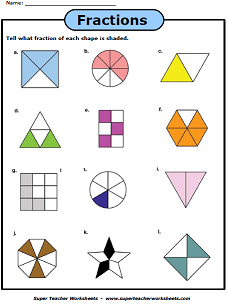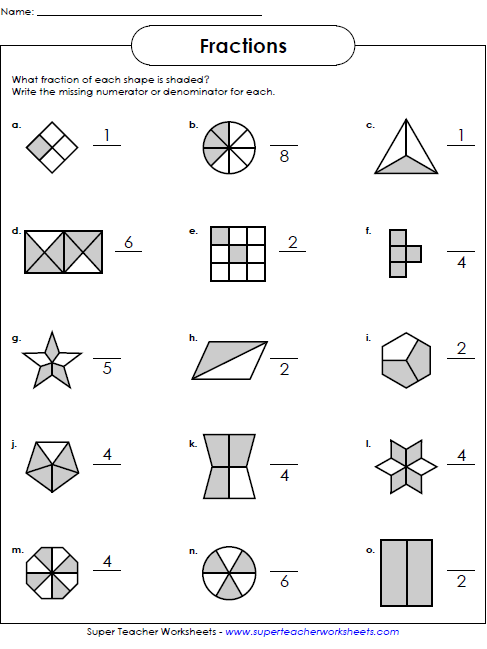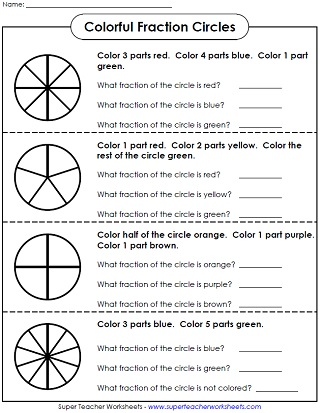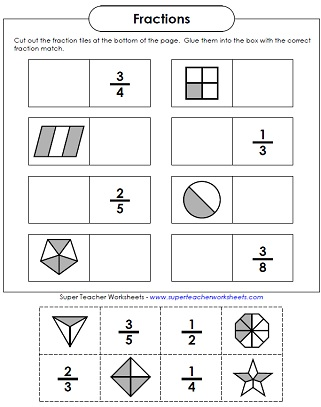My Account
Site Information